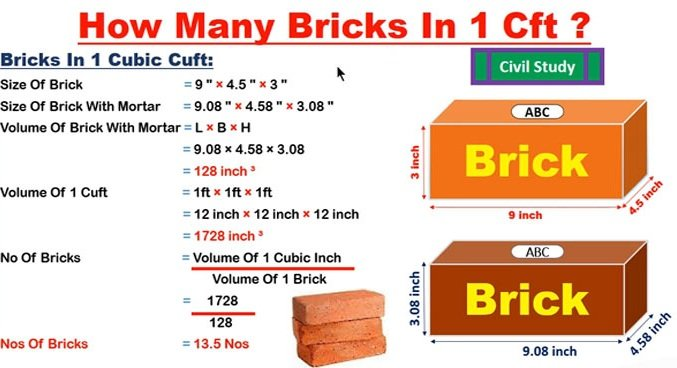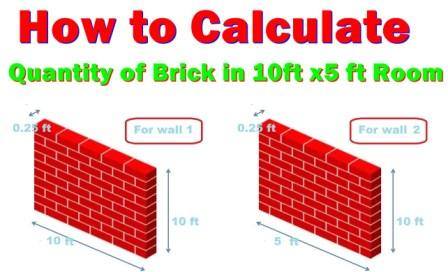Breaking News
Home / QA/QC Engineering / How to calculate the number of bricks in 1 CFT wall

# How to calculate the number of bricks in 1 CFT wall

## How to calculate the number of bricks in 1 CFT wall.

Hi, Civil Engineers, You might have knowledge about the quantity of bricks in one cubic meter wall. You may have a question what is the number of brick in  1 cft wall so this article is important for you.

In this article, I have explained how to calculate the quantity of bricks in 1 CFT wall.Here I have taken the field size bricks

## Quantity of Bricks in 1 CFT Wall

Length of Bricks in cft (L) = 9” = 0.75 ft

Width of Bricks in cft (W) = 5” = 0.41 ft

Thickness of Bricks in cft (T) =3” = 0.25 ft

Volume of Bricks in cft = L x W x T

= 0.75 x 0.41 x 0.25

= 0.076 ft3

2. ### How To Calculate The Cutting Length Of Circular Stirrup

Numbers of bricks in 1 cft = 1/(0.076) =13.15 bricks

Mortar covered 10 % space so

=13.15 x (10/100)

=1.315

Quantity of bricks = 13.15 – 1.315 =11.835 bricks

Adding the 5 % wastage of Bricks in Quantity of bricks = 11.835 x(5/100) = 0.591

Hence, the total numbers of bricks in 1 cft =11.835 + 0.591 = 12.426

We can say 12.5 numbers of bricks required in 1 cft.

Do you want to know how many numbers of Bricks required in the Area of Room?

## Quantity Bricks Required for 10 ft x 5ft Room Area

Let us use the above derivation by taking the room plan

Area of the room = 10 ft x 5 ft## For Wall ( 1)

Length of one side wall = 10 ft

Width of bricks = 0.25 ft

Height of the wall = 10 ft

Volume of wall in cft = 10 x 0.25 x 10 =25 ft3

For both side= 2 x 25 = 50 ft3 (for wall 1)

## For Wall (2)

Length of another wall = 5 ft

Width of bricks = 0.25 ft

Height of the wall = 10 ft

Volume of wall in cft = 5 x 0.25 x 10 = 12.5 ft3

For both sides = 2 x 12.5 = 25 ft3   ( for wall 2)

So total Volume of wall = Wall 1 + Wall 2

= 50 ft3 + 25 ft3   = 75 ft3

As per Above Derivation 1 cft =  12 .5 bricks

For 75 cft = 12.5 x 75 = 937.5 bricks required for making 10 ft x 5 ft  room.

## . Land Surveying & Architects

### THANKS.